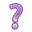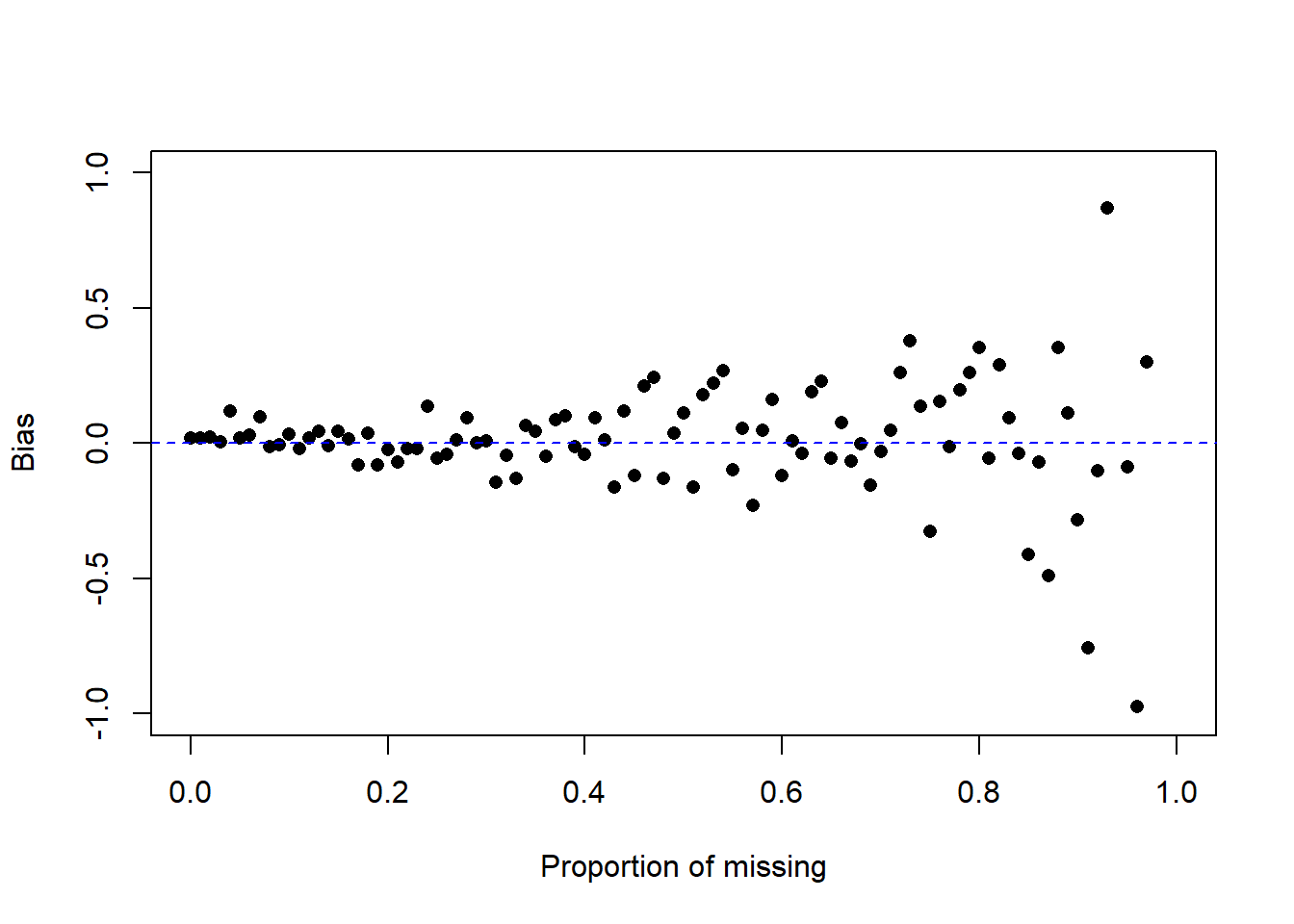## 18.3 Missing Data Mechanisms

Process by which some units observed, some units not observed

• Missing Completely at Random (MCAR): The probability that a data point is missing is completely unrelated (independent) of any observed and unobserved data or parameters.
• P(Y missing| X, Y) = P(Y missing)
• Missing at Random (MAR): The probability that a data point is missing is independent can be explained or modeled by other observed variables.
• P(Y missing|x, Y) = P(Y missing | X)
• Ex: Y = age, X = sex
- Pr (Y miss| X = male) = 0.2
- Pr (Y miss| X = female) = 0.3
- Males people are less likely to fill out an income survey - The missing data on income is related to gender - After accounting for gender the missing data is unrelated to income.
• Not missing at Random (NMAR): The probability that a data point is missing depends on the value of the variable in question.
• P(Y missing | X, Y) = P (Y missing|X, Y)
• Ex: Y = income, X = immigration status
• Richer person may be less willing to disclose income
• Undocumented immigrant may be less willing to disclose incomeWrite down an example of each.

Does it matter to inferences? Yes!

### 18.3.1 Demonstration via Simulation

What follows is just one method of approaching this problem via code. Simulation is a frequently used technique to understand the behavior of a process over time or over repeated samples.

#### 18.3.1.1 MCAR

1. Draw a random sample of size 100 from a standard Normal distribution (Z) and calculate the mean.
set.seed(456) # setting a seed ensures the same numbers will be drawn each time
z <- rnorm(100)
mean.z <- mean(z)
mean.z
##  0.1205748
1. Delete data at a rate of $$p$$ and calculate the complete case (available) mean.
• Sample 100 random Bernoulli (0/1) variables with probability $$p$$.
x <- rbinom(100, 1, p=.5)
• Find out which elements are are 1’s
delete.these <- which(x==1)
• Set those elements in z to NA.
z[delete.these] <- NA
• Calculate the complete case mean
mean(z, na.rm=TRUE)
##  0.1377305
2. Calculate the bias as the sample mean minus the true mean ($$E(\hat\theta) - \theta$$).
mean(z, na.rm=TRUE) - mean.z
##  0.01715565

How does the bias change as a function of the proportion of missing? Let $$p$$ range from 0% to 99% and plot the bias as a function of $$p$$.

calc.bias <- function(p){ # create a function to handle the repeated calculations
mean(ifelse(rbinom(100, 1, p)==1, NA, z), na.rm=TRUE) - mean.z
}

p <- seq(0,.99,by=.01)

plot(c(0,1), c(-1, 1), type="n", ylab="Bias", xlab="Proportion of missing")
points(p, sapply(p, calc.bias), pch=16)
abline(h=0, lty=2, col="blue")What is the behavior of the bias as $$p$$ increases? Look specifically at the position/location of the bias, and the variance/variability of the bias.

#### 18.3.1.3 NMAR: Pure Censoring

Consider a hypothetical blood test to measure a hormone that is normally distributed in the blood with mean 10$$\mu g$$ and variance 1. However the test to detect the compound only can detect levels above 10.

z <- rnorm(100, 10, 1)
y <- z
y[y<10] <- NA
mean(z) - mean(y, na.rm=TRUE)
##  -0.6850601

Did the complete case estimate over- or under-estimate the true mean?

When the data is not missing at random, the bias can be much greater.
Usually you don’t know the missing data mechanism.

Degrees of difficulty

• MCAR: is easiest to deal with.
• MAR: we can live with it.
• NMAR: most difficult to handle.

Evidence?

What can we learn from evidence in the data set at hand?

• May be evidence in the data rule out MCAR - test responders vs. nonresponders.
• Example: Responders tend to have higher/lower average education than nonresponders by t-test
• Example: Response more likely in one geographic area than another by chi-square test
• No evidence in data set to rule out MAR (although there may be evidence from an external data source)

What is plausible?

• Cochran example: when human behavior is involved, MCAR must be viewed as an extremely special case that would often be violated in practice
• Missing data may be introduced by design (e.g., measure some variables, don’t measure others for reasons of cost, response burden), in which case MCAR would apply
• MAR is much more common than MCAR
• We cannot be too cavalier about assuming MAR, but anecdotal evidence shows that it often is plausible when conditioning on enough information

Ignorable Missing

• If missing-data mechanism is MCAR or MAR then nonresponse is said to be “ignorable”.
• Origin of name: in likelihood-based inference, both the data model and missing-data mechanism are important but with MCAR or MAR, inference can be based solely on the data model, thus making inference much simpler
• Ignorability” is a relative assumption: missingness on income may be NMAR given only gender, but may be MAR given gender, age, occupation, region of the country